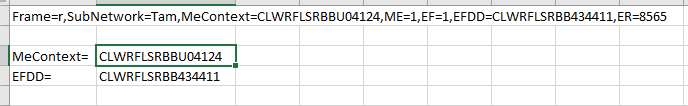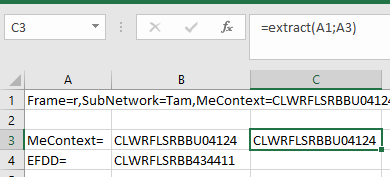# Excel formulas for extract (VBA)

Hi

I need excel formula or VBA.

I need extract between "MeContext=" and  ","  result should be in B1=CLWRFLSRBBU04124

and same need to be extract between "EFDD=" and "," result should be in C1=CLWRFLSRBB434411

A1=Frame=r,SubNetwork=Tam,MeContext=CLWRFLSRBBU04124,ME=1,EF=1,EFDD=CLWRFLSRBB434411,ER=8565
B1 = CLWRFLSRBBU04124
C1 = CLWRFLSRBB434411
###### Who is Participating?
I wear a lot of hats...

"The solutions and answers provided on Experts Exchange have been extremely helpful to me over the last few years. I wear a lot of hats - Developer, Database Administrator, Help Desk, etc., so I know a lot of things but not a lot about one thing. Experts Exchange gives me answers from people who do know a lot about one thing, in a easy to use platform." -Todd S.

Excel & VBA ExpertCommented:
Hi Vijay,

Try below in B1:
``````=MID(\$A1,SEARCH("MeContext=",\$A1)+10,SEARCH(",ME=",\$A1)-SEARCH("MeContext=",\$A1)-10)
``````
And below in C1:
``````=MID(\$A1,SEARCH("EFDD=",\$A1)+5,SEARCH(",ER=",\$A1)-SEARCH("EFDD=",\$A1)-5)
``````
Extract-Text-Between-Two-Text.xlsx
Author Commented:
Hi Shums,

Thanks you for your contribution, but  in formula comma (,) only is constant in position of  ",ME=" and ",ER=" , So ME= & ER= will be variable one.

So is possible to extract between  "MeContext=" and  "," only?
Excel & VBA ExpertCommented:
Hi Vijay,

You can extract 16 digit number after "MeContext=" with below formula:
``````=MID(\$A1,SEARCH("MeContext=",\$A1)+10,16)
``````
And 16 digit number after "EFDD=" with below formula:
``````=MID(\$A1,SEARCH("EFDD=",\$A1)+5,16)
``````
Extract-Text-Between-Two-Text_v2.xlsx
Commented:
Hi,

you can extract the text with this formula:
``````=SUBSTITUTE(LEFT(MID(\$A\$1,FIND(A3,\$A\$1)+LEN(A3),LEN(\$A\$1)-FIND(A3,\$A\$1)),FIND(",",MID(\$A\$1,FIND(A3,\$A\$1)+LEN(A3),LEN(\$A\$1)-FIND(A3,\$A\$1)))),",","")
``````

The string to be find have to end with a comma otherwise this formula doesn't work.Experts Exchange Solution brought to you by

Facing a tech roadblock? Get the help and guidance you need from experienced professionals who care. Ask your question anytime, anywhere, with no hassle.

Commented:
...and if like to use VBA....

``````Function extract(ori As String, toExtract As String) As String
Dim st() As String

st = Split(ori, ",")
For Each s In st
If InStr(1, s, toExtract) > 0 Then
extract = Split(s, "=")(1)
Exit Function
End If
Next
End Function
``````###### It's more than this solution.Get answers and train to solve all your tech problems - anytime, anywhere.Try it for free Edge Out The Competitionfor your dream job with proven skills and certifications.Get started today Stand Outas the employee with proven skills.Start learning today for free Move Your Career Forwardwith certification training in the latest technologies.Start your trial today
Microsoft Office

From novice to tech pro — start learning today.